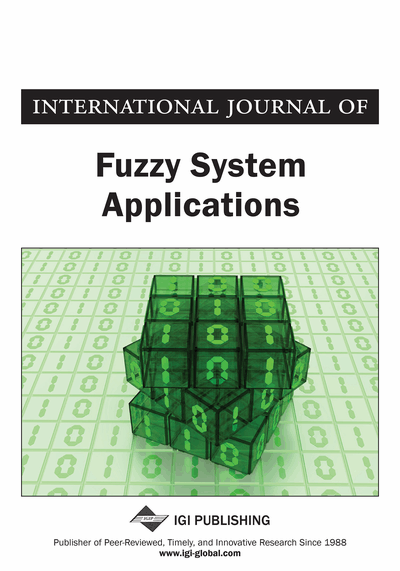# Multi-Fuzzy Complex Numbers and Multi-Fuzzy Complex Sets

Asit Dey (Department of Applied Mathematics with Oceanology and Computer Programming, Vidyasagar University, Midnapore, India) and Madhumangal Pal (Department of Applied Mathematics with Oceanology and Computer Programming, Vidyasagar University, Midnapore, India)
DOI: 10.4018/IJFSA.2015040102
Available
\$29.50
No Current Special Offers

## Abstract

This paper presents a method to construct more general fuzzy complex numbers and sets from ordinary fuzzy complex numbers by introduced the ordered sequences of membership functions. It is concluded that multi-fuzzy complex set is an extension of Buckley fuzzy complex set. Also, two types of multi-fuzzy complex numbers based on the forms and are investigated.
Article Preview
Top

## 1. Introduction

The elements of a crisp set are pairwise different but if we allow repeated occurrences of any element, then we get a mathematical structure. This mathematical structure is called multiset (Blizard, 1991). The numerous applications of multisets have found in mathematics and computer science. In addition multisets are used in concurrency theory (Nicola & Smolka 1996).

If we allow repeated occurrences of any element of a set integral number of times (includes a negative number of times), we get a structure that has been called hybrid set. Loeb (Loeb, 1992) introduced this mathematical structure and shown that one can use a hybrid set to describe the roots of a rational functions where elements that occur a positive number of times and describe the poles of a rational function where elements that occur a negative number of times.

Yager (Yager, 1985) developed the concept of fuzzy multisets from crisp multisets and it is a fuzzy subsets whose elements may occur more than once. The definition of fuzzy multisets given by Yager is as follows:

## Complete Article List

Search this Journal:
Reset
Volume 12: 1 Issue (2023)
Volume 11: 4 Issues (2022)
Volume 10: 4 Issues (2021)
Volume 9: 4 Issues (2020)
Volume 8: 4 Issues (2019)
Volume 7: 4 Issues (2018)
Volume 6: 4 Issues (2017)
Volume 5: 4 Issues (2016)
Volume 4: 4 Issues (2015)
Volume 3: 4 Issues (2013)
Volume 2: 4 Issues (2012)
Volume 1: 4 Issues (2011)
View Complete Journal Contents Listing